# Quizzes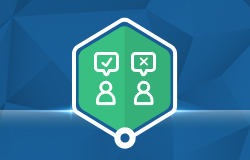Some courses in CoderZ offer formative quizzes!

These quizzes are usually at the end of a pack and can be used as an assessment tool, to give teachers a picture of how well their students understood the subjects taught in each lesson.

The quiz questions are multiple choice questions (either single-answer radio buttons or multi-answer checkboxes). Students receive immediate feedback per every question and a summary at the end of the quiz: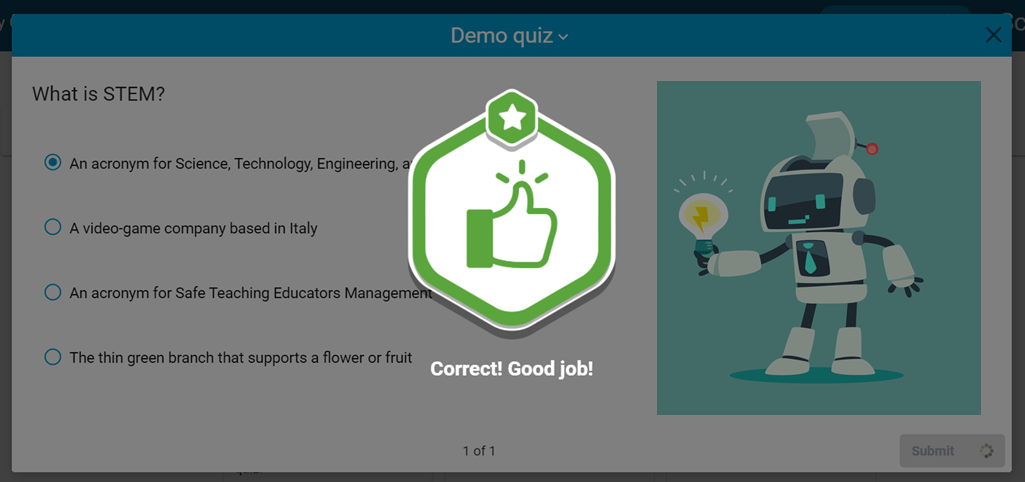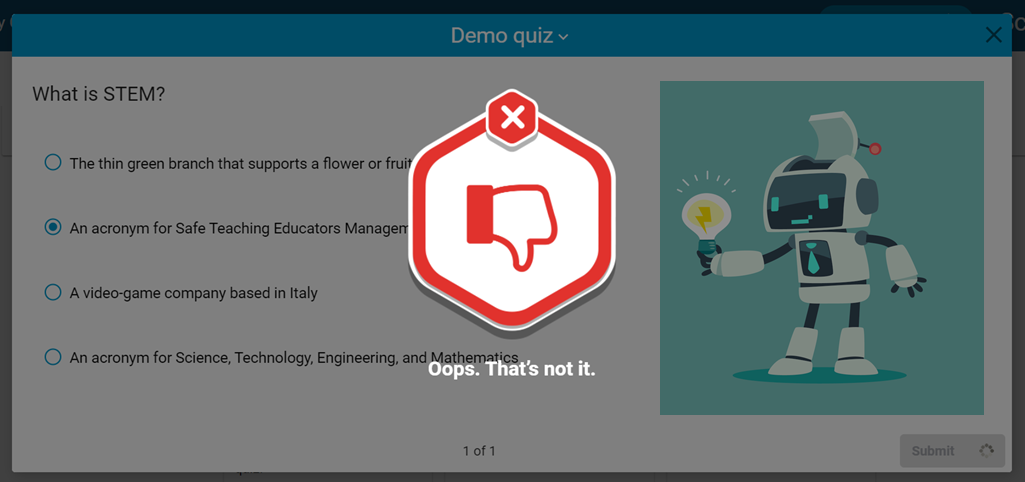Feedback for correct answer Feedback for incorrect answer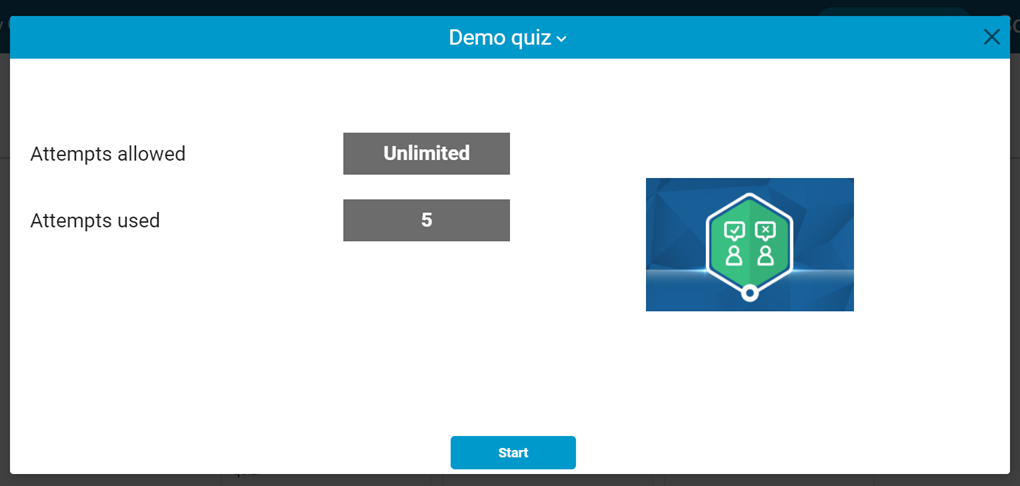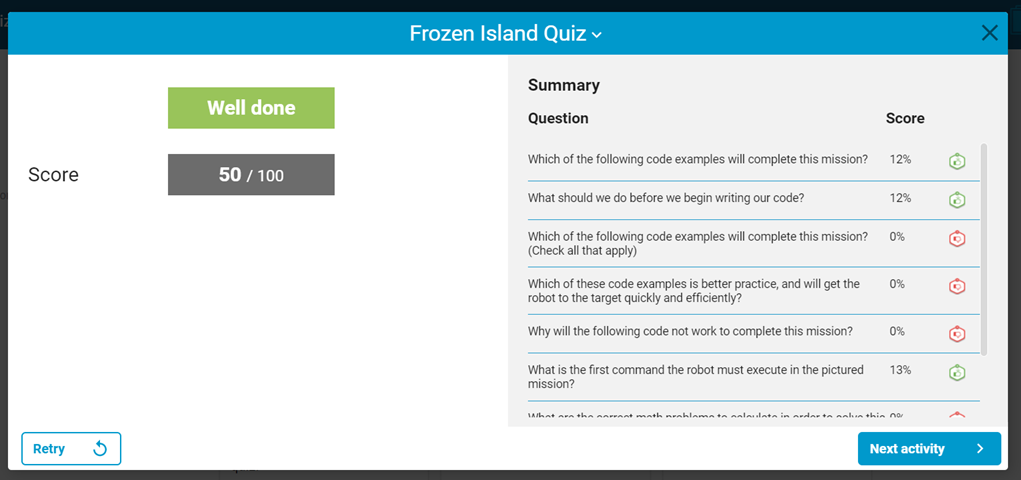Quiz start page Quiz summary

#### Teacher Review

Students may take and retake quizzes as many times as they wish, and teachers can see their performance on quizzes in the Class Heat Maps: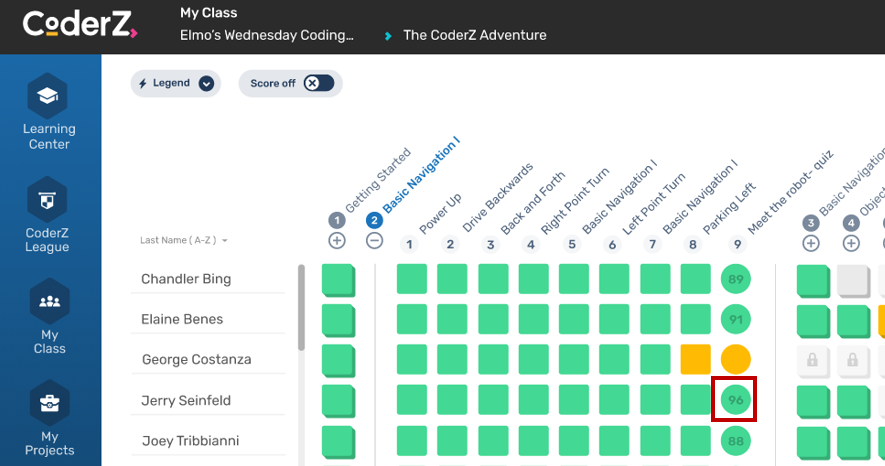By clicking a quiz tile on the Heat Map, teachers can review a student's answer choices for each quiz, starting with the summary: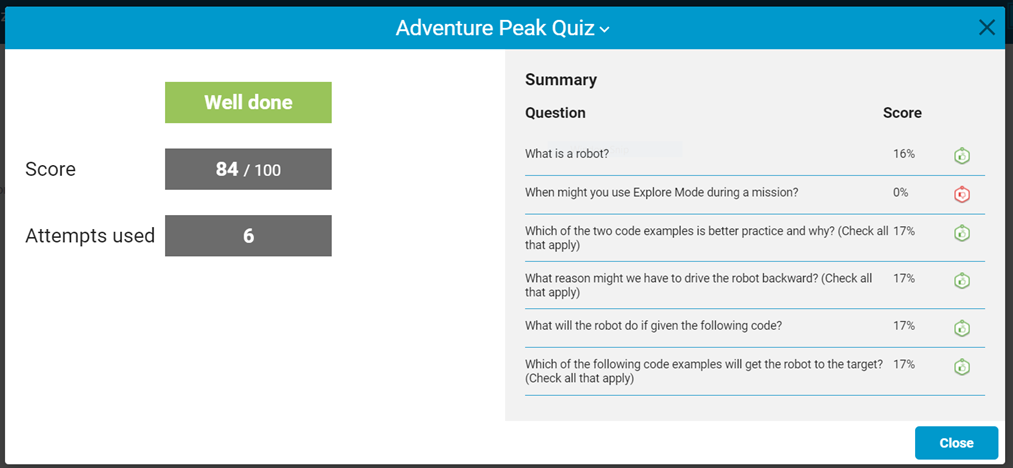By clicking any of the questions in the summary on the right, teachers will be taken to the question to see the student's answer choice for that question, and can then peruse the rest of the quiz questions by clicking the 'Next' and 'Previous' buttons, or else return to the summary.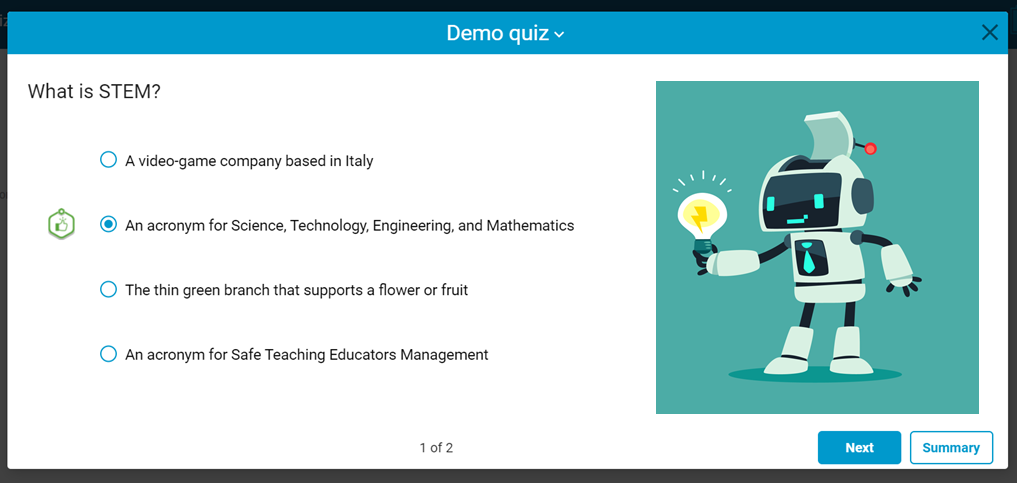Example of correct answer selected by student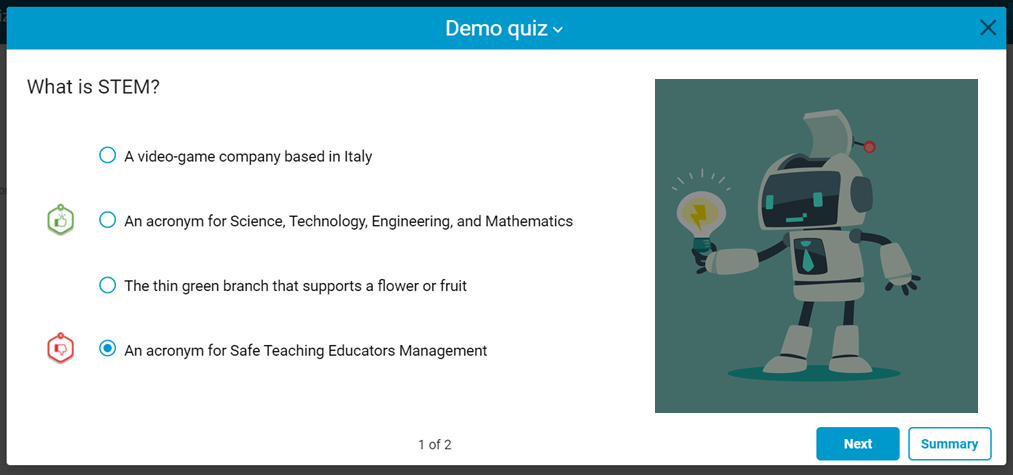Example of incorrect answer selected by student (bottom). The correct answer is marked as well (second option)